# CBSE Class 10 Science Sample Paper 2023 Set A

Read and download PDF of CBSE Class 10 Science Sample Paper 2023 Set A designed as per the latest curriculum and examination pattern for Class 10 issued by CBSE, NCERT and KVS. The latest Class 10 Science Sample Papers have been provided with solutions so that the students can solve these practice papers and then compare their answers. This will help them to identify mistakes and improvement areas in Science Standard 10 which they need to study more to get better marks in Grade 10 exams. After solving these guess papers also refer to solved Class 10 Science Question Papers available on our website to build strong understanding of the subject

## Sample Paper for Class 10 Science 2023 Pdf

Students can refer to the below Class 10 Science Sample Paper designed to help students understand the pattern of questions that will be asked in Grade 10 exams. Please download CBSE Class 10 Science Sample Paper 2023 Set A

### Science Class 10 Sample Paper 2023

SECTION – A

1. Which of the following a balanced equation for the following chemical reaction:(a) Zn + Ag(NO3)2 → Zn(NO3)2 + 2Ag
(b) 2Zn + 2AgNO3 → Zn(NO3)2 + 2Ag
(c) Zn + 2AgNO3 → Zn(NO3)2 + 2Ag
(d) Zn + AgNO3 → Zn(NO3)2 + Ag

2. Which of the following will turn phenolphthalein pink?
(a) NaOH(aq)
(b) HCl(aq)
(c) CH3COOH(aq)
(d) H2O

3. While studying the saponification reaction, what do you observe when you mix an equal amount of colourless vegetable oil and 20% aqueous solution of NaOH in a beaker?
(a) The colour of the mixture has become dark brown.
(b) A brisk effervescence is taking place in the beaker.
(c) The outer surface of the beaker has become hot.
(d) The outer surface of the beaker has become cold.

4. Why do we store silver chloride in dark-coloured bottles?
(a) To prevent precipitation of silver chloride
(b) To prevent decomposition of silver chloride
(c) To promote decomposition of silver chloride
(d) All of these

5. Which of the following gives the correct increasing order of the atomic radii of O, F and N ?
(a) O, F, N
(b) N, F, O
(c) O, N, F
(d) F, O, N

6. Identify X, Y and Z based on the basis of given information. X is a non-metal, which is an important constituent of our food. It forms two oxides Y and Z. Y is toxic and it causes suffocation and sometimes death. Z is responsible for global warming.
(a) X= C, Y= CO, Z=CO2
(b) X = S, Y= SO2, Z= SO3
(c) X=P, Y=P2O3, Z=P2O3
(d) X= O, Y = O2, Z = O3

7. Structural formulae of X and Y, which have the same molecular formula, C3H60 are :
(a) X = CH3CH2CHO, Y= CH3COCH3
(b) X = CH3OCH2CH3, Y= CH3COCH3
(c) X = CH3COCH3, Y = CH3COOH
(d) X= CH3CH2OCH3, Y = CH3CH2COOH

8. The correct sequence of reproductive stages seen in flowering plants is :
(a) Gametes, zygote, embryo, seedling
(b) Zygote, gametes, embryo, seedling
(c) Seedling, embryo, zygote, gametes
(d) Gametes, embryo, zygote, seedling

9. Mohan was confused and did not know how to draw the correct figure for geotropism. So, he has drawn three figures, as shown below.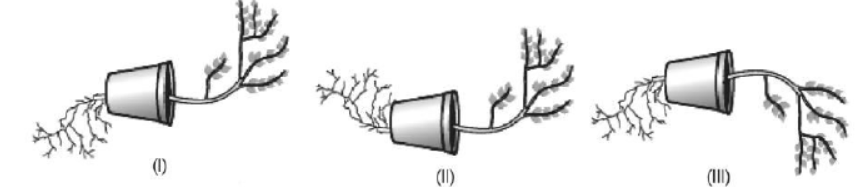Which appears more accurate and why?
(a) (I), (II)
(b) (I) only
(c) (II), (III)
(d) (II) only

10. Choose the correct statement about heart.
(a) The upper two chambers of human heart are called ventricles.
(b) The lower two chambers of human heart are called atrium.
(c) The chambers of the heart are separated by a partition called pericardium.
(d) The pulmonary artery carries deoxygenated blood from the right ventricle to the lungs

11. Choose the correct heading for the given below points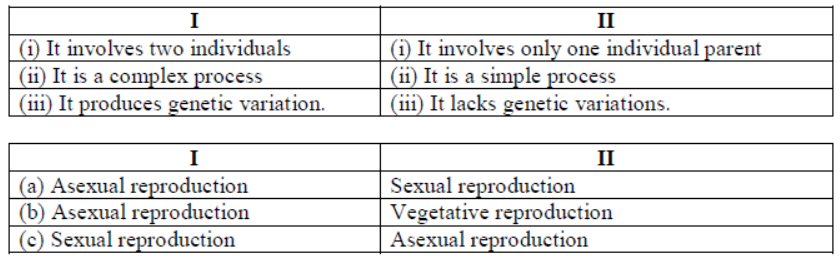12. Four students plotted the sketch of the patterns of magnetic field lines representing the magnetic field around a current carrying straight wire as shown in figures P, Q, R and S. Which one of the following sketches is correct?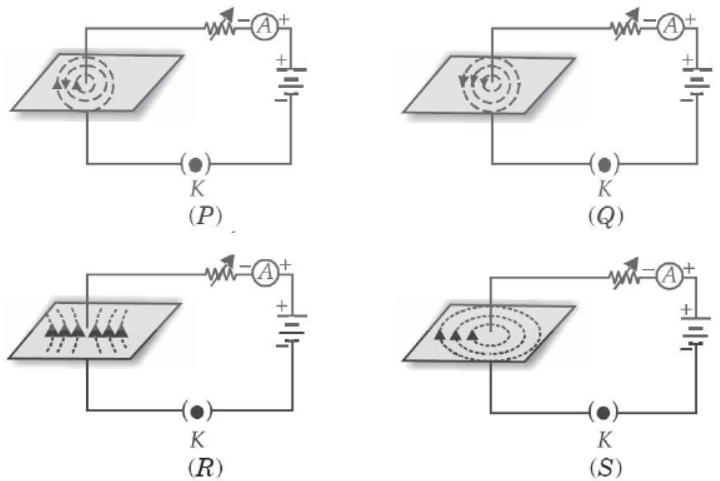(a) P
(b) Q
(c) R
(d) S

13. A pea plant is represented by Rr. This represents the
(a) Genetic composition of an individual
(b) Characteristics which are visible in an organism.
(c) Alternate form of genes
(d) None of these

14. The voltage-current (V-I) graph of a metallic conductor at two different temperatures T1 and T2 is shown:At which temperature is the resistance higher?
(a) T2
(b) T1
(c) Equal at both T2 and T1
(d) Cannot say

15. As per Michael Faraday, the forefinger, middle finger and thumb indicate the direction of:
(a) magnetic field, force and current respectively.
(b) magnetic field, current and force respectively.
(c) current, force and magnetic field respectively.
(d) force, magnetic field and current respectively.

16. An electron enters a magnetic field at right angles to it as shown in fig.
The direction of the force acting on the electron will be:(a) to the right
(b) to the left
(c) out of the page
(d) into the page

DIRECTION: In the question number 17 and 20, a statement of Assertion (A) is followed by a statement of Reason (R).
Choose the correct option
(a) Both assertion (A) and reason (R) are true and reason (R) is the correct explanation of assertion (A)
(b) Both assertion (A) and reason (R) are true and reason (R) is not the correct explanation of assertion (A)
(c) Assertion (A) is true but reason (R) is false.
(d) Assertion (A) is false but reason (R) is true.

17. Assertion (A): Fresh milk in which baking soda is added, takes a longer time to set as curd.
Reason (R): Baking soda decreases the pH value of fresh milk to below 6.

18. Assertion (a): The opening and closing of the pore is a function of the guard cells.
Reason (R): Stomatal pores are the site for exchange of gases by diffusion.

19. Assertion (A): Lipase help in emulsification of fats.
Reason (R): Lipase hydrolyses fats and oils.

20. Assertion (a): In a series circuit, the current is constant throughout the electric circuit.
Reason (R): All electric devices need equal currents to operate properly.

SECTION – B

21. Observe the given figure: What happens when the tube is heated?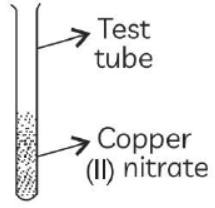(a) Write a balanced chemical equation of the reaction.
(b) Identify the brown gas X evolved.
Answer: (a) Balanced equation for the reaction taking place on heating copper (II) nitrate in a boiling tube is given below: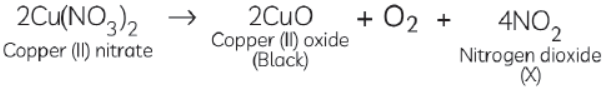(b) The brown gas X evolved is nitrogen dioxide gas (NO2).

22. Why does carbon become stable after sharing four electrons? What type of bond is formed by sharing?
Answer: The atomic number of carbons is 6 and its electronic configuration is 2, 4. It is tetravalent as it has 4 valence electrons. It can neither gain nor lose 4 electrons to acquire the nearest noble gas configuration. Only way is to share the four valence electrons with the electrons of other atoms. The type of bond formed by sharing of electrons is covalent bond.

OR

Why are covalent compounds being poor conductors of electricity? Why do covalent compounds have low melting and boiling points?
Answer: Covalent compounds are poor conductors of electricity as they do not have charged particles since the electrons are shared between atoms. Covalent compounds have low melting and boiling points as they have weak inter molecular forces due to which little energy is required in changing state by overcoming the force of attraction.

23. In birds and mammals, the left and right side of the heart are separated. Give reasons.
Answer: Ans: The separation keeps oxygenated and deoxygenated blood from mixing, allowing a highly efficient supply of oxygen to the body. This is useful in animals that have high energy needs (birds and mammals) which constantly use energy to maintain their body temperature.

24. Neha did not want to have a child. So, she went to a doctor who suggested her to adopt contraceptive methods. What are the reasons for adopting contraceptive methods?
(1) To avoid unwanted pregnancies.
(2) To keep the population of a country under control.
(3) To limit the number of children a couple wants to have.
(4) To maintain adequate gap between two consecutive children.
(5) To prevent the transmission of sexually transmitted diseases.

25. Define the term power of accommodation. Write the modification in the curvature of the eye lens which enables us to see the nearby objects clearly? Give relation between power and focal length.
Answer: Power of accommodation - Ability of eye lens to adjust its focal length. Curvature increases/lens becomes thick.
P = 1/f (in m) ⇒ P = 100/f (in cm)

OR

Why is the sun visible to us 2 minutes before actual sunrise and 2 minutes after actual sunset?
Answer: We are able to see the sun 2 minutes before actual sunrise and 2 minutes after actual sunset because sun rays undergo atmospheric refraction as they travel from an optically rarer medium to an optically denser medium when they enter the earth’s atmosphere due to which they are refracted downwards and hence appear to be above the horizon.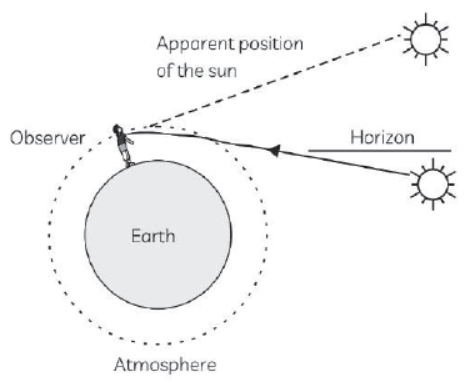26. DDT was sprayed in a lake to regulate breeding of mosquitoes. How would it affect the trophic levels in the following food chain associated with a lake? Justify your answer.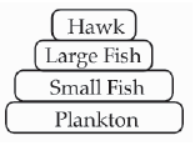Answer: DDT being a non- biodegradable pesticide will enter the food chain from the first trophic level i.e., Plankton.
Non - biodegradable pesticides accumulate progressively at each trophic level. This phenomenon is known as biological magnification
Hawk will have the highest level of pesticide.

SECTION – C

27. Based on the group valency of elements, write the molecular formula of the following compounds giving justification for each:
(a) Oxides of first group elements.
(b) Halides of the elements of group 13.
(c) Compounds formed when an element A of group 2 combines with an element B of group 17.
Answer: (a) Valency of group 1 element is 1. Valency of oxygen is 2.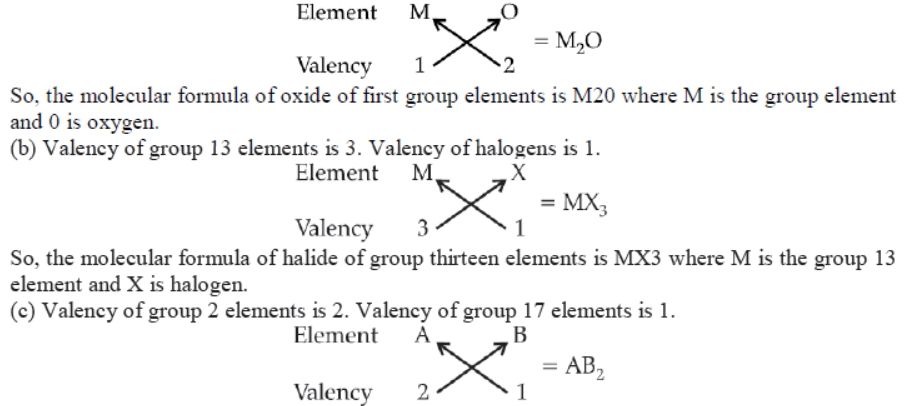So, the molecular formula is AB, where A is the group 2 element and B is the group 17 element.

28. Can two people with brown eyes have a blue-eyed baby? Explain.
Answer: Two people with brown eyes can have a blue-eyed baby but the chances are only 25% provided both the parents are heterozygous (Bb). Brown eye colour (b) is dominant and blue eye colour (b) is recessive.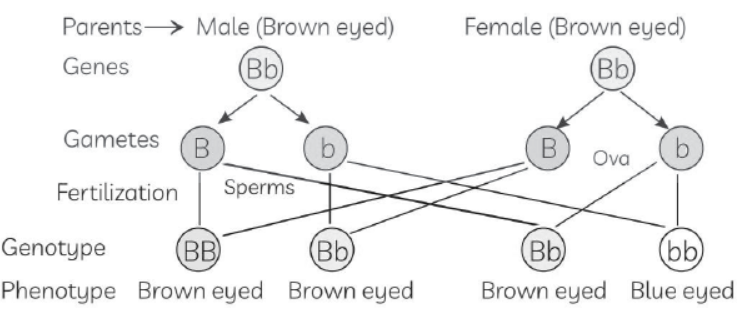Genotypic ratio: 1: 2: 1
Phenotypic ratio: 3: 1
The child who inherits ‘B’ chromosome even from one parent will have brown eyes. The child who inherits ‘b’ chromosomes from both the parents will have blue eyes so the chances of brown eyed parents to have blue eyed baby is only 25%.

29. Mention any three-information given by a chemical equation.
Answer: A balanced chemical equation tells:
(1) The number of atoms and molecules of reactants and products involved.
(2) The chemical formula of reactants and products involved.
(3) The catalyst involved in the reaction if any.

OR

State the law of conservation of mass as applicable in a chemical reaction. Illustrate with an example.
Answer: The law of conservation of mass states that matter can neither be created nor destroyed in a
chemical reaction. The mass of the products in a chemical reaction is equal to the mass of the
reactants. Example to illustrate the law of conservation of mass:
In the reaction CaCO3 → CaO + CO2,
The molecular mass of CaCO3 = 1 × 40 + 1 × 12 + 3 × 16 = 100 u
Molecular mass of CaO = 1 × 40 + 1 × 16 = 56 u and molecular mass of CO2 = 1 × 12 + 2 × 16
= 44 u
Mass of the reactant = 100 u
Mass of the product = 56 u + 44 u = 100 u.
As the mass of the reactant is equal to the mass of the products, law of conservation of mass is verified.

30. Derive an expression for electric energy consumed in a device in terms of V, I and t, where V is the potential difference applied to it, I is the current drawn by it and t is the time for which the current flows ?
Answer: Work done, W = QV
Also, P = W/t
Now, P = QV/t = QIR/t (∴ V = IR) ⇒ P = Q/t IR = I2R
Energy = P x t = I2Rt = V2/R2 Rt = V2/R(t)
where, V = voltage, R = resistance, t = time.

Please refer to attached file for CBSE Class 10 Science Sample Paper 2023 Set A